# Linear Regression Introduction

## Introduction

What is a Regression Problem?

A regression problem deals with the prediction of real or continuous-valued output variables such as Price. Our goal is to construct an efficient model that can predict the relationship between dependent and independent variables. Out of many different models, linear regression is the simplest one.

Below is a Flowchart for a better understanding of Regression Analysis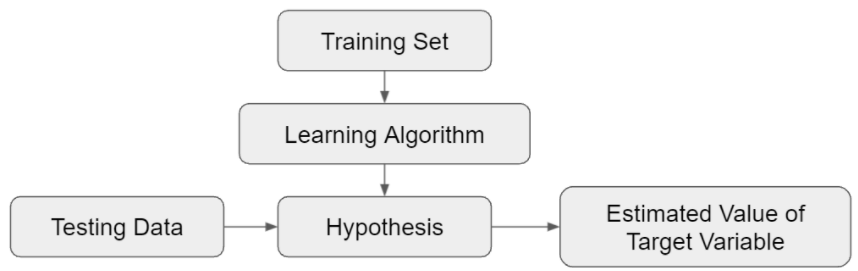## How Linear Regression works

Split the data into two sets, i.e., Training and Testing Data. Training data will train or teach the ML algorithm. In contrast, testing data will help validate the algorithm's predicted output and optimize it for better results.

There are two categories of variables in Linear Regression:

Dependent variable: The variable whose output we need to predict, also known as the Outcome variable.

Independent variable: It's the variable that we use to predict another variable's value. E.g., risk factors, predictors, or explanatory variables.

Linear Regression Analysis is the process of predicting the output of a variable based on values of other variables. The analysis aims to formulate a linear equation to predict the values of the dependent variable. The coefficients of the linear equation are estimated involving one or more independent variables.

For example, Below is a simple regression problem with a single dependent and independent variable (Y and x, respectively), the form of the equation would be:

Y = C0 + C1*x    (Hypothesis)

We obtain a straight line with a single input variable (x), but when we have multiple inputs (x), the line becomes a Plane or hyper-plane. The complexity of linear regression increases with an increase in the number of coefficients used in the Model.

The input and output variables are also known as features and target variables.

## Terminologies

Before moving on to the working of linear regression algorithm, let's understand some basic terminologies in a simple linear regression.

The best fit line for simple Linear Regression will be in the form of the equation given below.

Y = C0 + C1*x + e

Y: Dependent variable

C0: Y-axis intercept

C1: Slope of the line

e: Error in resultant prediction

Cost Function

The regression model aims to predict Y such that the error difference between the true value and the predicted value is minimum. So we keep on updating C0 and C1 to reach the best optimal value, which minimizes error to the least hence converting this search problem to a minimization problem.

Linear regression's cost function (J) is the Mean Squared Error between the True and Predicted value. We square the error difference, sum it over all the data points, and divide it with the total number of data points.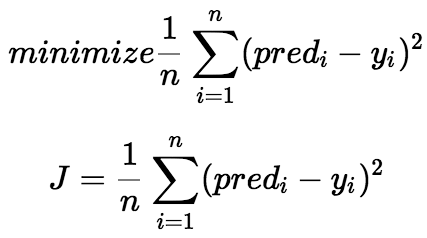Source: towardsdatascience.com

It's a method of updating C0 and C1 to minimize the Cost function (MSE). The idea is to start with random C0 and C1 and then iteratively change the values to reach the minimum cost.

## Linear Regression Algorithm

Estimating coefficients is done by analyzing and reducing errors between real and predicted values to get the optimal output equation.

Below is the explanation of the Least Squares Method.

Using Least Squares to fit a line to the Data

1. Assume we have the following plot for the Price of Real estate property against its size. There are a lot of factors in reality but let's take a more straightforward case.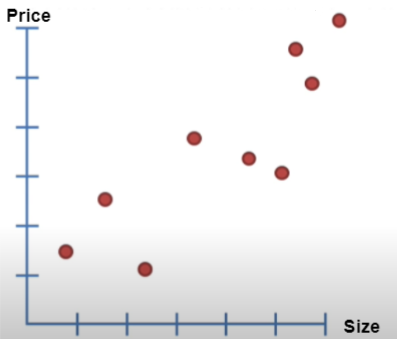2. A straight line is drawn through the data.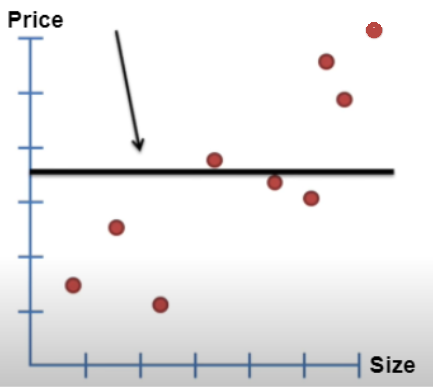3. Residual is calculated by measuring the distance from the line to the data. Next, Square the distances and add them up.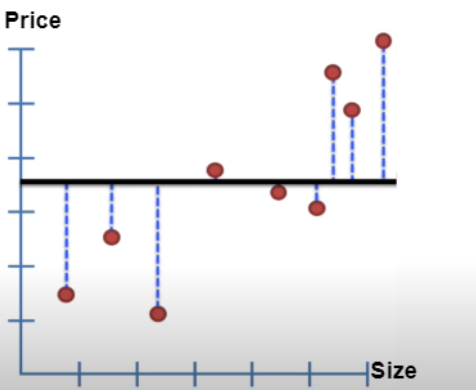4. The line is rotated, and the same process is repeated.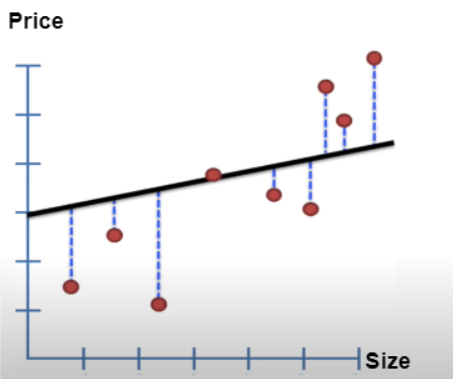5. After some rotations, the Sum of Squared Residual plot is plotted against their corresponding rotation, and The rotation with the least sum of squares is selected.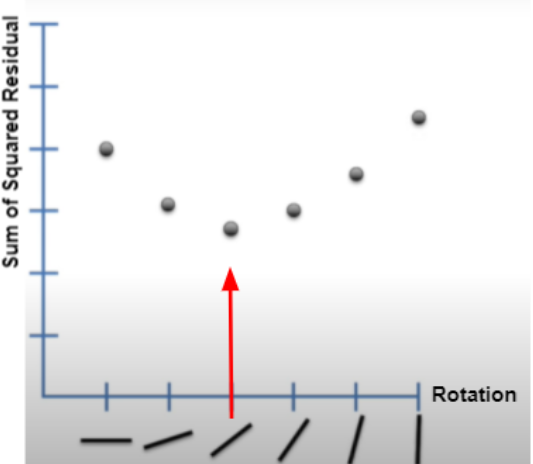6. Line with the least squared residual.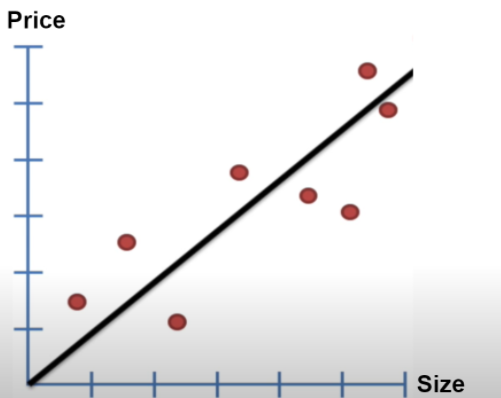Youtube Channel: StatQuest with Josh Starmer

The line with the least squared residual is obtained, giving us the linear equation's Y-axis intercept and slope. ( y = mx + c )

While Solving Regression Problems, We have a Hypothesis that consists of some parameters.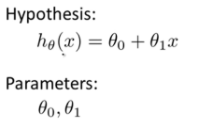We select a cost function, and our goal is to minimize the cost function.

You must watch this video for the conceptual implementation of the “Linear Regression Algorithm”.

## Implementation of Linear Regression in Python

We start by importing the necessary libraries such as pandas, numpy, model_selection from sklearn, and Data is loaded from a local CSV file.

Load data using the .loadtxt function and specify the path to the dataset. (Please select the path according to where the file is stored in your device if you are trying to run this code on your device)

``````import numpy as np
import matplotlib.pyplot as plt
from sklearn import model_selection

data = np.loadtxt("Linear Regression - Sheet1.csv", delimiter=",")
x = data[:, 0].reshape(-1, 1)
y = data[:, 1]``````

Train Test Split and estimation of Coefficients

``````from sklearn import model_selection
X_train, X_test, Y_train, Y_test = model_selection.train_test_split(x, y)
from sklearn.linear_model import LinearRegression
alg1 = LinearRegression()
alg1.fit(X_train, Y_train)``````

Plotting Results

``````import matplotlib.pyplot as plt
m = alg1.coef_
c = alg1.intercept_

x_line = np.arange(30,70,0.1)
y_line = m * x_line + c
plt.plot(x_line, y_line, "r")
train_1d = X_train.reshape(225)
plt.scatter(X_train, Y_train)
plt.show()``````

Output :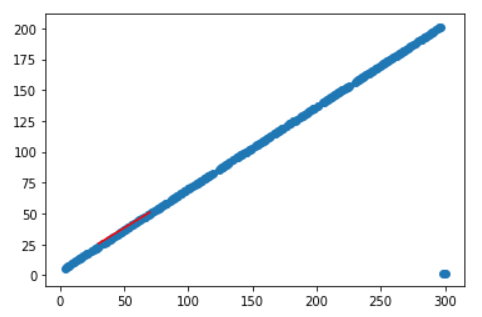## Preparing Data For Linear Regression

Below are some key points on How you must structure the data to get the best results from the Model.

• Linear Assumption: Linear regression assumes the relationship between your input and output to be linear. In some cases, when there are a lot of attributes, Data transformation might be required to make the relationship linear.
• Noise Removal: Data cleaning operations are recommended for better prediction of the output variable as linear regression can be very sensitive to outliers. e.g., removal of outliers in the output variable.
• Remove Collinearity. Linear regression can over-fit data when highly correlated input variables are present. Pairwise correlations can be calculated for the input data, and the most correlated ones can be removed.
• Rescale Inputs: Linear regression will make more reliable predictions if input variables are rescaled using standardization or normalization.

1. What are the four assumptions of Linear Regression?
Linearity: There exists a linear relationship between the dependent variable Y and the independent variable x.
Independence: There exists no relation between consecutive residuals in time-series data.
Normality: Residuals of the Model are normally distributed.
Equality of variance: The residuals have an equal variance for every level of x.

2. What is the difference between simple linear and multiple linear regression?
Simple linear regression has only one explanatory variable to predict the outcome of the dependent variable. In contrast, Multiple linear regression uses several explanatory or independent variables to predict the output variable.

3. Why do we square the error instead of using simple modulus?
We use square error to get the most negligible impact of values which contributes to the maximum error. Moreover, the squared error is differential while the absolute error is not, which makes the squared error more compatible with the Mathematical optimization techniques.

4. What is the limitation of Ordinary Least Squares (OLS)?
Ordinary Least Squares performs well with a smaller set of data. As the size of data grows, OLS becomes computationally expensive.

## Key Takeaways

This brings us to the end of this article, where we have explored the basics of Linear Regression for Machine Learning. I hope you are clear with all the material that has been provided in this article.

Linear Regression is a Basic algorithm that also serves as an entry point to Machine Learning, and every Machine Learning enthusiast must know about it. It's a handy and straightforward algorithm. I hope this article was helpful.